# Arelli

Arelli had 20 minutes to do a three-problem quiz. She spent 9 7/10 minutes on question A and 3 2/5 minutes on question B. How much time did she have left for question C?
Solve on paper to find the answer as a fraction.

c =  6.9 min

### Step-by-step explanation: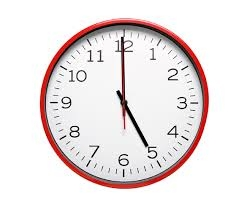Did you find an error or inaccuracy? Feel free to write us. Thank you!Tips to related online calculators
Do you want to convert time units like minutes to seconds?

## Related math problems and questions:

• Emily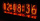Emily had 20 minutes to do a three-problem quiz. She spent 11 3/4 minutes on question A and 5 1/2 minutes on question B. How much time did she have left for question C?
• Quiz or testI have a quiz with 20 questions. Each question has 4 multiple choice answers, A, B, C, D. THERE IS NO WAY TO KNOW THE CORRECT ANSWER OF ANY GIVEN QUESTION, but the answers are static, in that if the "correct" answer to #1 = C, then it will always be equal
• DuringDuring a week, a student's spends 1/3 of her time in bed, 5/24 of her time in lesson and 1/8 of her time doing homeworks. What fraction of her time is left for doing other things?Nadia spent 1/4 of her money on a shirt and 2/5 of her money on new shoes. What fraction of Nadia´s money has been spent what fraction of her money is left?
• Fractions and mixed numerals(a) Convert the following mixed numbers to improper fractions. i. 3 5/8 ii. 7 7/6 (b) Convert the following improper fraction to mixed number. i. 13/4 ii. 78/5 (c) Simplify these fractions to their lowest terms. i. 36/42 ii. 27/45 2. evaluate following ex
• JonahJonah has an 8-pound bag of potting soil. He divides it evenly among 5 flowerpots. How much soil is in each pot? Show your answer as a fraction or mixed number. Solve this problem any way you choose.
• Bus vs train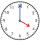Milada took the bus and the journey took 55 minutes. Jarmila was 1h 20 min by train. They arrived in Prague at the same time 10h45 min. At what time did each have to go out?
• Free timeAnita had 2 1/4 hours of free time in the afternoon. She spent 2/3 of her free time playing sports and watched TV for 30 minutes. How much time did she have left to read the book? Express the result as fractions of hours and numbers in minutes.
• Each year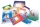Each year, Mr. Baumann purchases enough paper for his 16 students. If each student will use 3/4 pack of paper, how many packs of paper will he need to purchase? Answer as a mixed number or as a whole number.
• When EmilyWhen Emily arrived home from school, she spent 8 minutes eating a snack. Then, she spent 15 minutes playing with her dog and 32 minutes doing her chores. Finally, Emily spent 45 minutes finishing her homework. How long had Emily been home when she finishe
• Bus linesAt 5.00 in the morning, four buses left the terminal at the same time. The first bus has an interval of 15 minutes, the second 20 minutes, the third 25 minutes, and the fourth 45 minutes. How many minutes will all four buses leave the terminal at the same
• Evaluate 17Evaluate 2x+6y when x=- 4/5 and y=1/3. Write your answer as a fraction or mixed number in simplest form.
• WithdrawalIf I withdrew 2/5 of my total savings and spent 7/10 of that amount. What fraction do I have in left in my savings?Why does 1 3/4 + 2 9/10 equal 4.65? How do you solve this?The sum of 3 mixed numbers is 20 13/15. two of the numbers are 6 1/3 and 7 5/6. what is the third number?Write a real world problem involving the multiplication of a fraction and a whole number with a product that is between 8 and 10 then solve the problem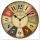Determine the difference in minutes: T1 = 2 3/20 h T2 = 2.3 h